### Home > A2C > Chapter 10 > Lesson 10.2.4 > Problem10-111

10-111.
1. Jenny and Gina were trying to solve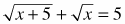. They knew they would have to square the equation to get rid of the radicals, but when they tried it, they got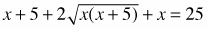, which did not look much easier to deal with. Gina had an idea. Homework Help ✎

2. Use Jenny and Gina's method to solve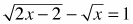.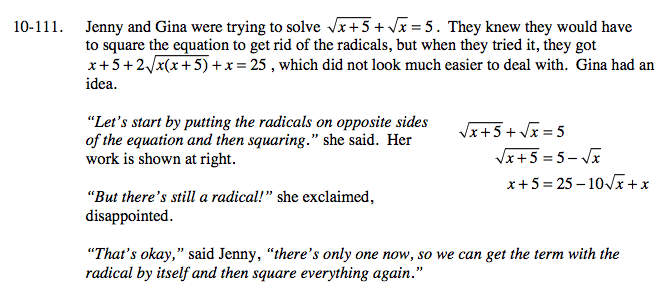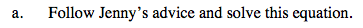$\sqrt{x+5}=5-\sqrt{x}$

$\sqrt{x+5}^{2}=(5-\sqrt{x})^{2}$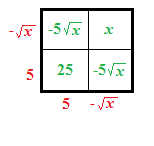$x+5=25-10\sqrt{x}+x$

x = 4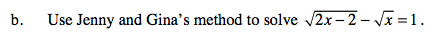See part (a).

x = 1 or x = 9
One of these does not check.